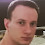# Decimal to Arbitrary Numeral System Conversion in C#

Developers often need to convert decimal numbers to binary, octal, hexadecimal or another numeral system. As this is a common task, there are many examples over the Internet how to do it. You can easily find a lot of decimal to binary, decimal to octal, decimal to hexadecimal, etc. converters but it is hard to find a more generalized converter that can convert a decimal number to any other numeral system. That is what I'm going to show you in this article. The presented implementation can convert any decimal number to an arbitrary numeral system with radix from 2 to 36.

Using the method is very simple:

``````long number = 123456789;
Console.WriteLine("Decimal: " + number.ToString());
Console.WriteLine("Binary : " + DecimalToArbitrarySystem(number,  2));
Console.WriteLine("Octal  : " + DecimalToArbitrarySystem(number,  8));
Console.WriteLine("Hex    : " + DecimalToArbitrarySystem(number, 16));
Console.WriteLine("Base 36: " + DecimalToArbitrarySystem(number, 36));

// This example displays the following output:
// Decimal: 123456789
// Binary : 111010110111100110100010101
// Octal  : 726746425
// Hex    : 75BCD15
// Base 36: 21I3V9``````

Here's the C# source code of the decimal to arbitrary numeral system converter:

``````/// <summary>
/// Converts the given decimal number to the numeral system with the
/// specified radix (in the range [2, 36]).
/// </summary>
/// <param name="decimalNumber">The number to convert.</param>
/// (in the range [2, 36]).</param>
/// <returns></returns>
public static string DecimalToArbitrarySystem(long decimalNumber, int radix)
{
const int BitsInLong = 64;
const string Digits = "0123456789ABCDEFGHIJKLMNOPQRSTUVWXYZ";

throw new ArgumentException("The radix must be >= 2 and <= " +
Digits.Length.ToString());

if (decimalNumber == 0)
return "0";

int index = BitsInLong - 1;
long currentNumber = Math.Abs(decimalNumber);
char[] charArray = new char[BitsInLong];

while (currentNumber != 0)
{
int remainder = (int)(currentNumber % radix);
charArray[index--] = Digits[remainder];
}

string result = new String(charArray, index + 1, BitsInLong - index - 1);
if (decimalNumber < 0)
{
result = "-" + result;
}

return result;
}``````

The article Arbitrary to Decimal Numeral System Conversion in C# demonstrates how to implement the reverse function that converts any number from an arbitrary to decimal numeral system.

1.Do you have a reverse function? This is exactly what I need, but I'll need the back to decimal function as well.

1.Yes, I've created an article for the reverse function, too. You can find it here: Arbitrary to Decimal Numeral System.

2.Pavel, what is the license for the code DecimalToArbitrarySystem. I may like to use it inside an open source project.

1.Hi Frederic, I guess you are talking about the Jint open source project (I looked at your blog). Feel free to use this code snippet in your project. I will greatly appreciate a link on your blog or GitHub page to this article, as well as a simple comment in the source code with a link to this article, too.

3.Really good helpful piece of code. Thanks ! We see more example of base32/64 encoding for binary representation than radix converter.